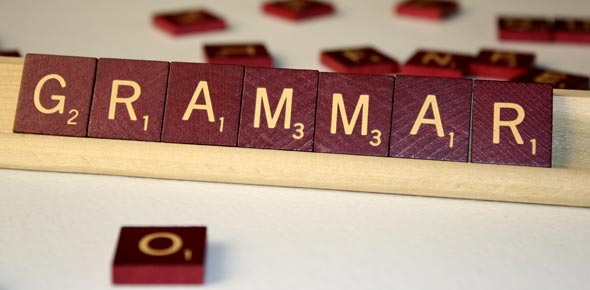# Test-2 Positive And Negative Questions (Am, Is, Are)

100 Questions | Total Attempts: 621SettingsCreate your own QuizThis is a test that queries various random questions that are designed to test your knowledge on grammar and positive and negative questions to be specific. It is not as easy as you may think but just try it out to see how you will do.

• 1.
.....................................you married?
• A.

Is

• B.

Are

• C.

Am

• D.

Be

• 2.
............................she a student?
• A.

Is

• B.

Are

• C.

Am

• D.

Be

• 3.
.......................you from LOndon?
• A.

Am

• B.

Is

• C.

Are

• D.

Be

• 4.
.............................you hungry?
• A.

Are

• B.

Is

• C.

Am

• D.

Be

• 5.
...........................she thirsty?
• A.

Am

• B.

Be

• C.

Are

• D.

Is

• 6.
...........................you tired?
• A.

Am

• B.

Are

• C.

Is

• D.

Be

• 7.
• A.

Be

• B.

Is

• C.

Am

• D.

Are

• 8.
• A.

Am

• B.

Is

• C.

Are

• D.

Be

• 9.
......................he at home?
• A.

Be

• B.

Am

• C.

Are

• D.

Is

• 10.
• A.

Are

• B.

Be

• C.

Is

• D.

Am

• 11.
...................your father at home?
• A.

Are

• B.

Am

• C.

Is

• D.

Be

• 12.
.....................he hungry?
• A.

Am

• B.

Is

• C.

Be

• D.

Are

• 13.
......................it cold today?
• A.

Is

• B.

Are

• C.

Am

• D.

Be

• 14.
..................it dark now?
• A.

Be

• B.

Are

• C.

Am

• D.

Is

• 15.
..........................you a teacher?
• A.

Is

• B.

Are

• C.

Am

• D.

Be

• 16.
• A.

Is

• B.

Be

• C.

Are

• D.

Am

• 17.
Where ...................... the car?
• A.

Is

• B.

Are

• C.

Am

• D.

Be

• 18.
• A.

Are

• B.

Be

• C.

Is

• D.

Am

• 19.
What ........................his name?
• A.

Be

• B.

Are

• C.

Am

• D.

Is

• 20.
Where ...................... your girl?
• A.

Be

• B.

Am

• C.

Are

• D.

Is

• 21.
..................your parents at home?
• A.

Are

• B.

Be

• C.

Am

• D.

Is

• 22.
........................you late?
• A.

Is

• B.

Am

• C.

Be

• D.

Are

• 23.
What color ........................... your car?
• A.

Be

• B.

Are

• C.

Am

• D.

Is

• 24.
How .............................Susan?
• A.

Am

• B.

Be

• C.

Are

• D.

Is

• 25.
Who ..................this man?
• A.

Are

• B.

Be

• C.

Is

• D.

Am

Related TopicsBack to top
×

Wait!
Here's an interesting quiz for you.